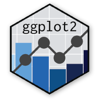Show Sidebar Hide Sidebar# geom_density in ggplot2

Add a smooth density estimate calculated by stat_density with ggplot2 and R. Examples, tutorials, and code.

### New to Plotly?

Plotly's R library is free and open source!
You can set up Plotly to work in online or offline mode.
We also have a quick-reference cheatsheet (new!) to help you get started!

### Version Check

Version 4 of Plotly's R package is now available!
Check out this post for more information on breaking changes and new features available in this version.

``````library(plotly)
packageVersion('plotly')
``````
``````##  '4.9.1'
``````

### Basic Density Plot

``````library(plotly)

library(ggplot2)
set.seed(1234)

dfGamma = data.frame(nu75 = rgamma(100, 0.75),
nu1 = rgamma(100, 1),
nu2 = rgamma(100, 2))

dfGamma = stack(dfGamma)

p <- ggplot(dfGamma, aes(x = values)) +
stat_density(aes(group = ind, color = ind),position="identity",geom="line")

p <- ggplotly(p)

p
``````

### Density & Facet

``````library(plotly)

require(plyr)
dd<-data.frame(matrix(rnorm(144, mean=2, sd=2),72,2),c(rep("A",24),rep("B",24),rep("C",24)))
colnames(dd) <- c("x_value", "Predicted_value",  "State_CD")

dd <- data.frame(
predicted = rnorm(72, mean = 2, sd = 2),
state = rep(c("A", "B", "C"), each = 24)
)

grid <- with(dd, seq(min(predicted), max(predicted), length = 100))
normaldens <- ddply(dd, "state", function(df) {
data.frame(
predicted = grid,
density = dnorm(grid, mean(df\$predicted), sd(df\$predicted))
)
})

p <- ggplot(dd, aes(predicted))  +
geom_density() +
geom_line(aes(y = density), data = normaldens, colour = "red") +
facet_wrap(~ state)

p <- ggplotly(p)

p
``````

### Multiple Density Plot

``````library(plotly)

carrots <- data.frame(length = rnorm(100000, 6, 2))
cukes <- data.frame(length = rnorm(50000, 7, 2.5))

#Now, combine your two dataframes into one.  First make a new column in each.
carrots\$veg <- 'carrot'
cukes\$veg <- 'cuke'

#and combine into your new data frame vegLengths
vegLengths <- rbind(carrots, cukes)

p <- ggplot(vegLengths, aes(length, fill = veg)) + geom_density(alpha = 0.2)

p <- ggplotly(p)

p
``````

### Stacked Density Plot

``````library(plotly)
set.seed(123)

df <- data.frame(x <- rchisq(1000, 5, 10),
group <- sample(LETTERS[1:5], size = 1000, replace = T))

p <- ggplot(df, aes(x, fill = group)) +
geom_density(alpha = 0.5, position = "stack") +
ggtitle("stacked density chart")

p <- ggplotly(p)

p
``````